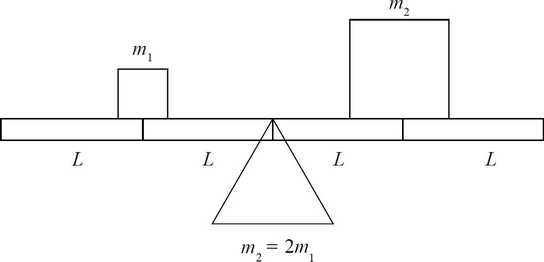# AP Physics 1 Question 326: Answer and Explanation

### Test Information

Question: 3264. As it is, the system above is not balanced. Which of the following changes would NOT balance the system so that there is 0 net torque? Assume the plank has no mass of its own.

• A. Adding a mass equal to m2 on the far left side and a mass equal to m1 and on the far right side
• B. Stacking both masses directly on top of the fulcrum
• C. Moving the fulcrum a distance L/3 to the right
• D. Moving both masses a distance L/3 to the left